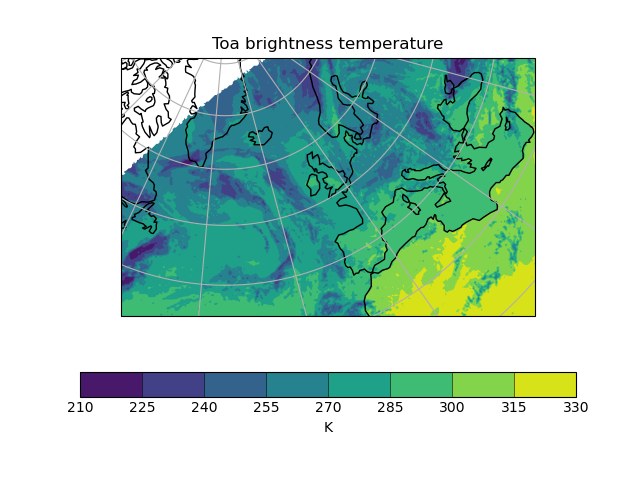# Example of a Polar Stereographic Plot#

Demonstrates plotting data that are defined on a polar stereographic projection.```import matplotlib.pyplot as plt

import iris
import iris.plot as iplt
import iris.quickplot as qplt

def main():
file_path = iris.sample_data_path("toa_brightness_stereographic.nc")
qplt.contourf(cube)
ax = plt.gca()
ax.coastlines()
ax.gridlines()
iplt.show()

if __name__ == "__main__":
main()
```

Total running time of the script: ( 0 minutes 1.525 seconds)

Gallery generated by Sphinx-Gallery